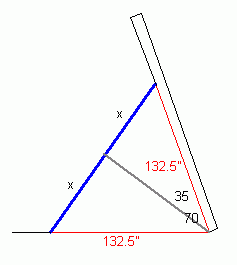SEARCH HOMEMath Central Quandaries & QueriesQuestion from james: I am trying to adjust the placement of a hydraulic cylinder that raises a dump bed up from the frame of a truck. How long would the cylinder (height of a triangle) have to be to raise the bed to a 70 degree angle? The base from pivot to cylinder is 132.5 inches.Hi James.

Unless I misunderstand, the cylinder will not be straight up and down when it is fully extended, right?

Then I think you have an isosceles triangle with common side lengths of 132.5 inches and shared vertex of 70 degrees.The third side of an isosceles triangle can be found using the sine trigonometric function. In the diagram below, I drew an imaginary line from the hinge to the midpoint of the cylinder. This divides the angle into two 35 degree right triangles, with the hypotenuse of 132.5 inches. So the length of x in the right triangle is the 132.5" times the sine of 35.

Cylinder length = 2x = 2 (132.5) sin(70) = 152".

Cheers,
Stephen La Rocque.Math Central is supported by the University of Regina and The Pacific Institute for the Mathematical Sciences.